Next: 5. Dielectric Degradation and Up: 4. Characterization of Interfaces Previous: 4.2 DCIV Method

# 4.3 Capacitance-Voltage Characteristics

The capacitance-voltage (CV) measurement can be used to determine the interface quality . During the CV measurement the device is swept from accumulation to inversion while constantly measuring the capacitance as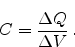(4.8)

Figure 4.12(a) depicts the simulated CV characteristics of a p-type MOSFET at different degradation levels. The density-of-states is assumed constant. It is a low frequency simulation (kHz) where the substrate carriers are in thermal equilibrium and follow the gate voltage change. An increase in the interface trap density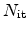is followed by deformation of the characteristics as the traps can dynamically be charged and discharged. An introduction of fixed oxide charges shifts the flat-band voltage and therefore the whole CV characteristics (Figure 4.12(b)) as predicted by Equation 2.31.

Figure 4.12: Capacitance-voltage simulation demonstrating the influence of (a) interface trapsand (b) fixed oxide charges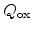. While interface traps lead to deformation of the CV curve, the introduction of oxide charges shifts the flat-band voltage and therefore the CV characteristics.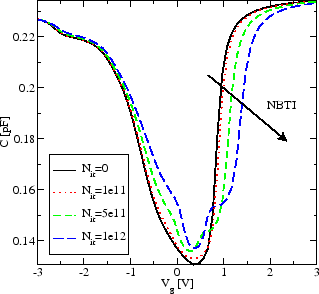Interface traps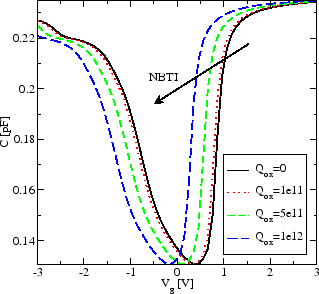Oxide charges

Increasing the frequency changes the CV results . As the interface traps are not fast enough and can therefore not follow the gate voltage variation their impact on the CV characteristics is reduced as shown in Figure 4.13. For very high frequencies the inversion layer cannot build up fast enough and the capacitance is drastically reduced.

Of major importance in the CV results is the trap density-of-states. Figure 4.14 gives the simulation results for different trap energy distributions. The exponential distribution has most traps close to the band edge. These traps can be filled and emptied even close to flat-band voltage conditions more easily and lead to an increase in the capacitance.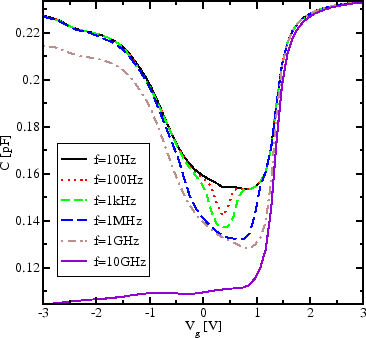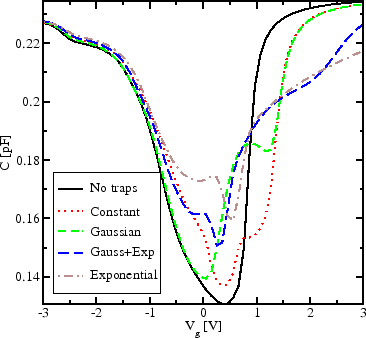Next: 5. Dielectric Degradation and Up: 4. Characterization of Interfaces Previous: 4.2 DCIV Method

R. Entner: Modeling and Simulation of Negative Bias Temperature Instability